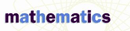# Numbers: Abundant, Deficient, Perfect, and Amicable - Research Article from Macmillan Science Library: Mathematics

This encyclopedia article consists of approximately 4 pages of information about Numbers.
 This section contains 973 words (approx. 4 pages at 300 words per page) View a FREE sampleWhat are "abundant" numbers? Why would such numbers be called plentiful? "Abundant" is a strange way to describe a number, and equally strange are descriptions such as "deficient," "perfect," and "amicable." But these descriptions of numbers came about because the ancient Greek mathematicians were intrigued by certain characteristics of positive integers.

The Greeks discovered, for example, that some numbers are equal to the sum of their divisors; for instance, 6 is equal to the sum of its proper divisors 3, 2, and 1. (Although 6 is a divisor of 6, it is not considered a "proper" divisor.) Greek mathematicians discovered a sense of balance or perfection in such numbers, and labeled them "perfect."

As an extension of the idea of perfect numbers, the concept of "abundant" and "deficient" numbers emerged. If the sum of the proper divisors of a number is greater than the...

 This section contains 973 words (approx. 4 pages at 300 words per page) View a FREE sample